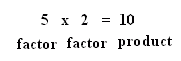Email us to get an instant 20% discount on highly effective K-12 Math & English kwizNET Programs!

#### Online Quiz (WorksheetABCD)

Questions Per Quiz = 2 4 6 8 10

### Grade 2 - Mathematics7.1 What is Multiplication?

 Explanation: Multiplication is repeated addition. Instead of adding many times, we just multiply the number by the number of times it appears.Here we can add 2 five times: 2 + 2 + 2 + 2 +2 = 10 You could also say 5 rows of two Or you can write that as a multiplication problemThe numbers that are multiplied 5 and 2 are called factors and 10 is called the product. Example: 3+3+3+3 This can be written as 3 x 4 In the above 3 and 4 are called factors and 12 is called the product. Answer : 12 Directions: Answer the following questions. Also write 20 multiplication facts of your own.
 Q 1: When two numbers are multiplied the answer is calledsumproductdifference Question 2: This question is available to subscribers only!